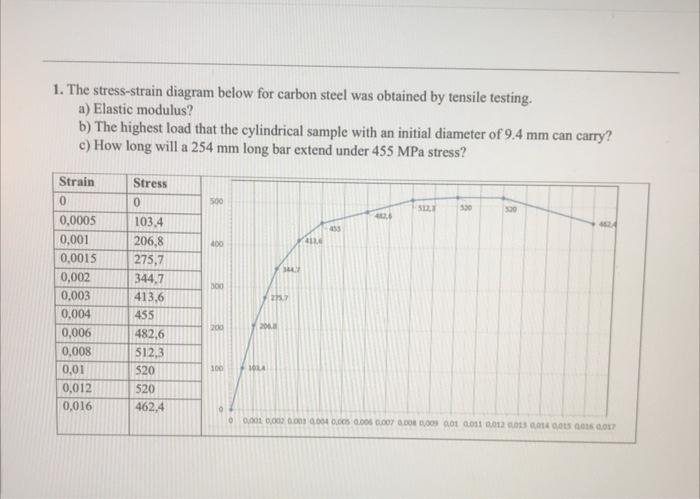# (Solved): Can you please explain and answer my question about Materials Science 1. The stress-strain diagram b ...1. The stress-strain diagram below for carbon steel was obtained by tensile testing. a) Elastic modulus? b) The highest load that the cylindrical sample with an initial diameter of $$9.4 \mathrm{~mm}$$ can carry? c) How long will a $$254 \mathrm{~mm}$$ long bar extend under $$455 \mathrm{MPa}$$ stress?

We have an Answer from Expert